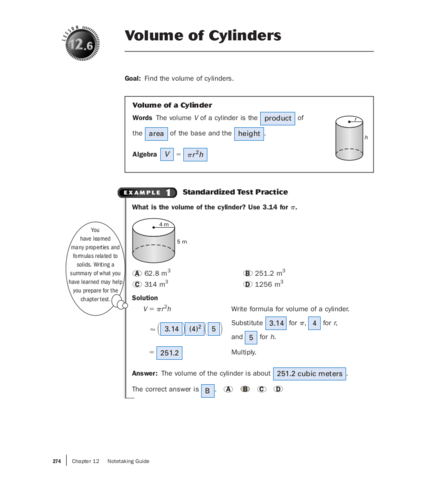# Volume of a CylinderContributed by:The volume V of a cylinder is of the area of the base and the height. Algebra V= πr^2h. Also, this pdf includes Comparing Volumes of Cylinders and Standardized Test Practice.
1. N
SO
Volume of Cylinders
LES
Goal: Find the volume of cylinders.
Volume of a Cylinder
Words The volume V of a cylinder is the product of r
the area of the base and the height .
h
Algebra V  πr 2h
EXAMPLE 1 Standardized Test Practice
What is the volume of the cylinder? Use 3.14 for π.
4m
You
have learned 5m
many properties and
formulas related to
solids. Writing a
summary of what you A 62.8 m3 B 251.2 m3
have learned may help C 314 m3 D 1256 m3
you prepare for the
chapter test. Solution
V  πr 2h Write formula for volume of a cylinder.
Substitute 3.14 for π, 4 for r,
  3.14  (4)2  5 
and 5 for h.
 251.2 Multiply.
Answer: The volume of the cylinder is about 251.2 cubic meters .
The correct answer is B . A B C D
274 | Chapter 12 Notetaking Guide
2. EXAMPLE 2 Comparing Volumes of Cylinders
Tomato Sauce Carlos found two cans of tomato sauce in the pantry. One
can has a diameter of 4 inches and a height of 5 inches. The second one
has a diameter of 3 inches and a height of 6 inches. Which can has the
greater volume?
Solution
WATCH OUT!
1. Find the radius of each can, which is 4 in. 3 in.
Make sure to use the
half of the diameter.
in the formula for 4
Can 1: r  2  2 in. 5 in.
6 in.
volume of a cylinder.
3
Can 2: r  2  1.5 in.
2. Find the volume of each can. Use 3.14 for π.
Can 1: Can 2:
V  πr h 2
V  πr 2h
  3.14  (2)2  5    3.14  (1.5)2  6 
 62.8 in.3  42.39 in.3
Answer: Can 1 has the greater volume.
EXAMPLE 3 Finding the Radius of a Cylinder
A cylinder has a height of 12 feet and a volume of 3768 cubic feet. Find
the radius of the cylinder. Use 3.14 for π.
V  πr 2h Write formula for volume of a cylinder.
Need
Substitute 3768 for V, 3.14 for π,
help with solving 3768   3.14 r 2 12 
equations using and 12 for h.
square roots? See
page 579 of your 3768  37.68r 2 Multiply.
textbook.
100  r 2 Divide each side by 37.68 .
  r
100 Take positive square root of each side.
10  r Evaluate square root.